# Building A Neural Net from Scratch Using R - Part 2

by Akshaj Verma

Akshaj is a budding deep learning researcher who loves to work with R. He has worked as a Research Associate at the Indian Institute of Science and as a Data Scientist at KPMG India.

In the previous post, we went through the dataset, the pre-processing involved, train-test split, and talked in detail about the architecture of the model. We started build our neural net chunk-by-chunk and wrote functions for initializing parameters and running forward propagation.

In the this post, we’ll implement backpropagation by writing functions to calculate gradients and update the weights. Finally, we’ll make predictions on the test data and see how accurate our model is using metrics such as Accuracy, Recall, Precision, and F1-score. We’ll compare our neural net with a logistic regression model and visualize the difference in the decision boundaries produced by these models.

Let’s continue by implementing our cost function.

### Compute Cost

We will use Binary Cross Entropy loss function (aka log loss). Here, $$y$$ is the true label and $$\hat{y}$$ is the predicted output.

$cost = - 1/N\sum_{i=1}^{N} y_{i}\log(\hat{y}_{i}) + (1 - y_{i})(\log(1 - \hat{y}_{i}))$

The computeCost() function takes as arguments the input matrix $$X$$, the true labels $$y$$ and a cache. cache is the output of the forward pass that we calculated above. To calculate the error, we will only use the final output A2 from the cache.

computeCost <- function(X, y, cache) {
m <- dim(X)
A2 <- cache$A2 logprobs <- (log(A2) * y) + (log(1-A2) * (1-y)) cost <- -sum(logprobs/m) return (cost) } cost <- computeCost(X_train, y_train, fwd_prop) cost ##  0.693 ### Backpropagation Now comes the best part of this all: backpropagation! We’ll write a function that will calculate the gradient of the loss function with respect to the parameters. Generally, in a deep network, we have something like the following.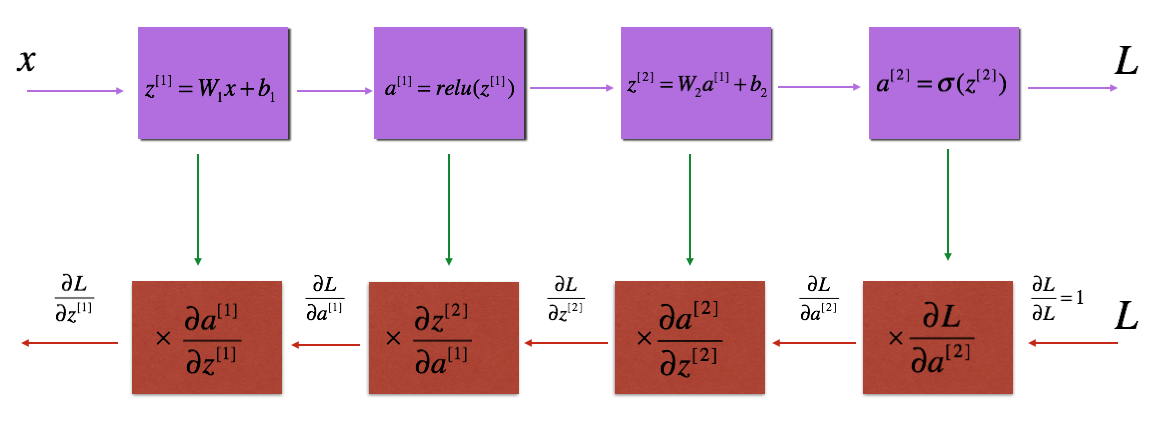The above figure has two hidden layers. During backpropagation (red boxes), we use the output cached during forward propagation (purple boxes). Our neural net has only one hidden layer. More specifically, we have the following: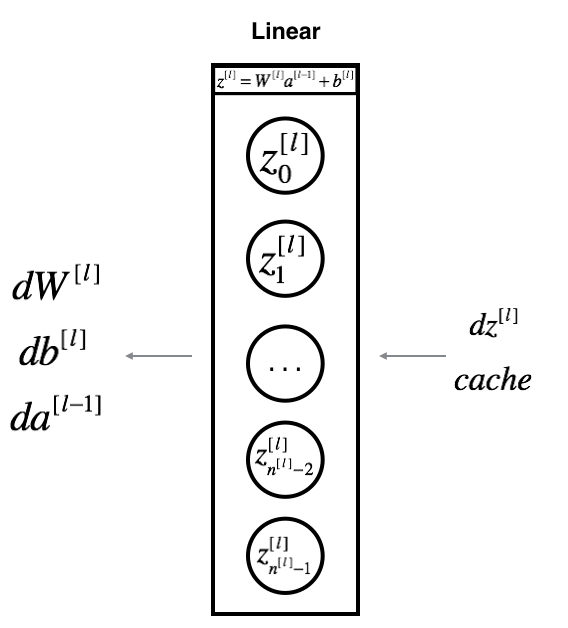To compute backpropagation, we write a function that takes as arguments an input matrix X, the train labels y, the output activations from the forward pass as cache, and a list of layer_sizes. The three outputs $$(dW^{[l]}, db^{[l]}, dA^{[l-1]})$$ are computed using the input $$dZ^{[l]}$$ where $$l$$ is the layer number. We first differentiate the loss function with respect to the weight $$W$$ of the current layer. $dW^{[l]} = \frac{\partial \mathcal{L} }{\partial W^{[l]}} = \frac{1}{m} dZ^{[l]} A^{[l-1] T} \tag{8}$ Then we differentiate the loss function with respect to the bias $$b$$ of the current layer. $db^{[l]} = \frac{\partial \mathcal{L} }{\partial b^{[l]}} = \frac{1}{m} \sum_{i = 1}^{m} dZ^{[l](i)}\tag{9}$ Once we have these, we calculate the derivative of the previous layer with respect to $$A$$, the output + activation from the previous layer. $dA^{[l-1]} = \frac{\partial \mathcal{L} }{\partial A^{[l-1]}} = W^{[l] T} dZ^{[l]} \tag{10}$ Because we only have a single hidden layer, we first calculate the gradients for the final (output) layer and then the middle (hidden) layer. In other words, the gradients for the weights that lie between the output and hidden layer are calculated first. Using this (and chain rule), gradients for the weights that lie between the hidden and input layer are calculated next. Finally, we return a list of gradient matrices. These gradients tell us the the small value by which we should increase/decrease our weights such that the loss decreases. Here are the equations for the gradients. I’ve calculated them for you so you don’t differentiate anything. We’ll directly use these values - • $$dZ^{} = A^{} - Y$$ • $$dW^{} = \frac{1}{m} dZ^{}A^{^T}$$ • $$db^{} = \frac{1}{m}\sum dZ^{}$$ • $$dZ^{} = W^{^T} * g^{'} Z^{}$$ where $$g$$ is the activation function. • $$dW^{} = \frac{1}{m}dZ^{}X^{T}$$ • $$db^{} = \frac{1}{m}\sum dZ^{}$$ If you would like to know more about the math involved in constructing these equations, please see the references below. backwardPropagation <- function(X, y, cache, params, list_layer_size){ m <- dim(X) n_x <- list_layer_size$n_x
n_h <- list_layer_size$n_h n_y <- list_layer_size$n_y

A2 <- cache$A2 A1 <- cache$A1
W2 <- params$W2 dZ2 <- A2 - y dW2 <- 1/m * (dZ2 %*% t(A1)) db2 <- matrix(1/m * sum(dZ2), nrow = n_y) db2_new <- matrix(rep(db2, m), nrow = n_y) dZ1 <- (t(W2) %*% dZ2) * (1 - A1^2) dW1 <- 1/m * (dZ1 %*% t(X)) db1 <- matrix(1/m * sum(dZ1), nrow = n_h) db1_new <- matrix(rep(db1, m), nrow = n_h) grads <- list("dW1" = dW1, "db1" = db1, "dW2" = dW2, "db2" = db2) return(grads) } As you can see below, the shapes of the gradients are the same as their corresponding weights i.e. W1 has the same shape as dW1 and so on. This is important because we are going to use these gradients to update our actual weights. back_prop <- backwardPropagation(X_train, y_train, fwd_prop, init_params, layer_size) lapply(back_prop, function(x) dim(x)) ##$dW1
##  4 2
##
## $db1 ##  4 1 ## ##$dW2
##  1 4
##
## $db2 ##  1 1 ### Update Parameters From the gradients calculated by the backwardPropagation(), we update our weights using the updateParameters() function. The updateParameters() function takes as arguments the gradients, network parameters, and a learning rate. Why a learning rate? Because sometimes the weight updates (gradients) are too large and because of that we miss the minima completely. Learning rate is a hyper-parameter that is set by us, the user, to control the impact of weight updates. The value of learning rate lies between $$0$$ and $$1$$. This learning rate is multiplied with the gradients before being subtracted from the weights.The weights are updated as follows where the learning rate is defined by $$\alpha$$. • $$W^{} = W^{} - \alpha * dW^{}$$ • $$b^{} = b^{} - \alpha * db^{}$$ • $$W^{} = W^{} - \alpha * dW^{}$$ • $$b^{} = b^{} - \alpha * db^{}$$ Updated parameters are returned by updateParameters() function. We take the gradients, weight parameters, and a learning rate as the input. grads and params are calculated above while we choose the learning_rate. updateParameters <- function(grads, params, learning_rate){ W1 <- params$W1
b1 <- params$b1 W2 <- params$W2
b2 <- params$b2 dW1 <- grads$dW1
db1 <- grads$db1 dW2 <- grads$dW2
db2 <- grads$db2 W1 <- W1 - learning_rate * dW1 b1 <- b1 - learning_rate * db1 W2 <- W2 - learning_rate * dW2 b2 <- b2 - learning_rate * db2 updated_params <- list("W1" = W1, "b1" = b1, "W2" = W2, "b2" = b2) return (updated_params) } As we can see, the weights still maintain their original shape. This means we’ve done things correctly till this point. update_params <- updateParameters(back_prop, init_params, learning_rate = 0.01) lapply(update_params, function(x) dim(x)) ##$W1
##  4 2
##
## $b1 ##  4 1 ## ##$W2
##  1 4
##
## $b2 ##  1 1 ## Train the Model Now that we have all our components, let’s go ahead write a function that will train our model. We will use all the functions we have written above in the following order. 1. Run forward propagation 2. Calculate loss 3. Calculate gradients 4. Update parameters 5. Repeat This trainModel() function takes as arguments the input matrix X, the true labels y, and the number of epochs. 1. Get the sizes for layers and initialize random parameters. 2. Initialize a vector called cost_history which we’ll use to store the calculated loss value per epoch. 3. Run a for-loop: • Run forward prop. • Calculate loss. • Update parameters. • Replace the current parameters with updated parameters. This function returns the updated parameters which we’ll use to run our model inference. It also returns the cost_history vector. trainModel <- function(X, y, num_iteration, hidden_neurons, lr){ layer_size <- getLayerSize(X, y, hidden_neurons) init_params <- initializeParameters(X, layer_size) cost_history <- c() for (i in 1:num_iteration) { fwd_prop <- forwardPropagation(X, init_params, layer_size) cost <- computeCost(X, y, fwd_prop) back_prop <- backwardPropagation(X, y, fwd_prop, init_params, layer_size) update_params <- updateParameters(back_prop, init_params, learning_rate = lr) init_params <- update_params cost_history <- c(cost_history, cost) if (i %% 10000 == 0) cat("Iteration", i, " | Cost: ", cost, "\n") } model_out <- list("updated_params" = update_params, "cost_hist" = cost_history) return (model_out) } Now that we’ve defined our function to train, let’s run it! We’re going to train our model, with 40 hidden neurons, for 60000 epochs with a learning rate of 0.9. We will print out the loss after every 10000 epochs. EPOCHS = 60000 HIDDEN_NEURONS = 40 LEARNING_RATE = 0.9 train_model <- trainModel(X_train, y_train, hidden_neurons = HIDDEN_NEURONS, num_iteration = EPOCHS, lr = LEARNING_RATE) ## Iteration 10000 | Cost: 0.3724 ## Iteration 20000 | Cost: 0.4081 ## Iteration 30000 | Cost: 0.3273 ## Iteration 40000 | Cost: 0.4671 ## Iteration 50000 | Cost: 0.4479 ## Iteration 60000 | Cost: 0.3074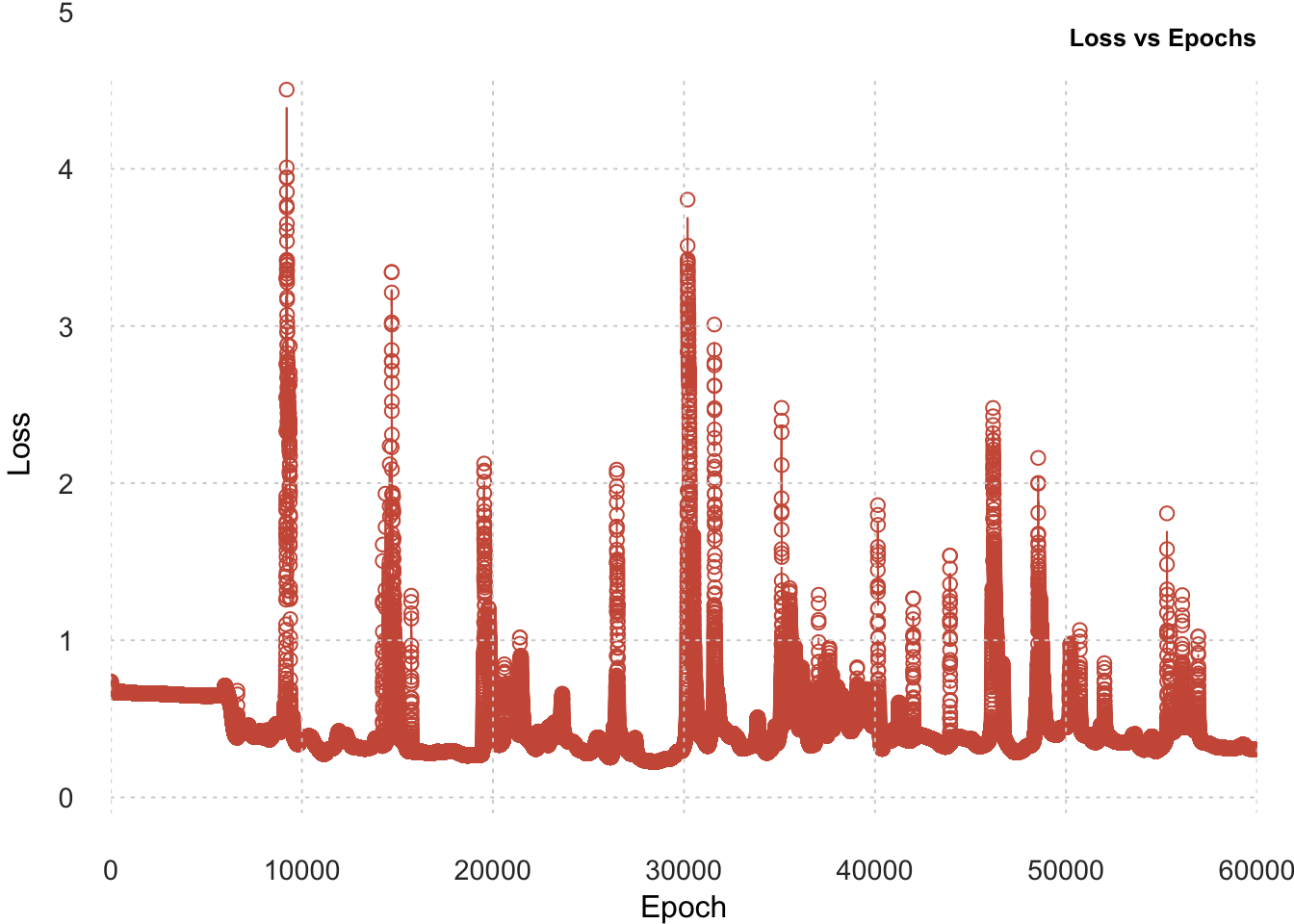## Logistic Regression Before we go ahead and test our neural net, let’s quickly train a simple logistic regression model so that we can compare its performance with our neural net. Since, a logistic regression model can learn only linear boundaries, it will not fit the data well. A neural-network on the other hand will. We’ll use the glm() function in R to build this model. lr_model <- glm(y ~ x1 + x2, data = train) lr_model ## ## Call: glm(formula = y ~ x1 + x2, data = train) ## ## Coefficients: ## (Intercept) x1 x2 ## 0.51697 0.00889 -0.05207 ## ## Degrees of Freedom: 319 Total (i.e. Null); 317 Residual ## Null Deviance: 80 ## Residual Deviance: 76.4 AIC: 458 Let’s now make generate predictions of the logistic regression model on the test set. lr_pred <- round(as.vector(predict(lr_model, test[, 1:2]))) lr_pred ##  1 1 0 1 0 1 1 1 0 1 0 1 0 1 0 0 0 1 1 1 1 1 0 0 0 0 1 1 1 0 1 0 1 1 1 1 1 1 ##  1 1 1 1 0 0 1 0 0 0 1 1 0 1 1 0 1 1 0 1 1 0 1 0 0 0 1 0 1 0 1 1 0 1 1 1 0 0 ##  1 1 1 0 ## Test the Model Finally, it’s time to make predictions. To do that - 1. First get the layer sizes. 2. Run forward propagation. 3. Return the prediction. During inference time, we do not need to perform backpropagation as you can see below. We only perform forward propagation and return the final output from our neural network. (Note that instead of randomly initializing parameters, we’re using the trained parameters here. ) makePrediction <- function(X, y, hidden_neurons){ layer_size <- getLayerSize(X, y, hidden_neurons) params <- train_model$updated_params
fwd_prop <- forwardPropagation(X, params, layer_size)
pred <- fwd_prop\$A2

return (pred)
}

After obtaining our output probabilities (Sigmoid), we round-off those to obtain output labels.

y_pred <- makePrediction(X_test, y_test, HIDDEN_NEURONS)
y_pred <- round(y_pred)

Here are the true labels and the predicted labels.

## Neural Net:
##  1 0 1 0 0 0 0 0 0 0 1 1 0 1 0 0 1 0 1 1 0 1 0 0 0 0 1 0 1 0 1 1 0 0 1 0 1 1 1 0 1 1 0 0 1 1 0 1 1 0 1 0 1 0 0 0 1 0 1 0 0 0 1 1 0 0 1 1 1 1 1 0 1 1 0 0 1 1 0 1
## Ground Truth:
##  0 0 1 0 0 0 0 0 1 0 1 1 0 0 0 1 1 0 1 0 0 1 0 1 0 0 0 0 1 1 1 1 0 1 0 0 1 1 1 0 1 0 0 1 1 1 0 1 1 0 1 0 1 0 0 0 1 0 0 1 0 0 1 1 0 0 0 1 1 0 0 0 1 1 0 0 1 1 0 1
## Logistic Reg:
##  1 1 0 1 0 1 1 1 0 1 0 1 0 1 0 0 0 1 1 1 1 1 0 0 0 0 1 1 1 0 1 0 1 1 1 1 1 1 1 1 1 1 0 0 1 0 0 0 1 1 0 1 1 0 1 1 0 1 1 0 1 0 0 0 1 0 1 0 1 1 0 1 1 1 0 0 1 1 1 0

### Decision Boundaries

In the following visualization, we’ve plotted our test-set predictions on top of the decision boundaries.

#### Neural Net

As we can see, our neural net was able to learn the non-linear decision boundary and has produced accurate results.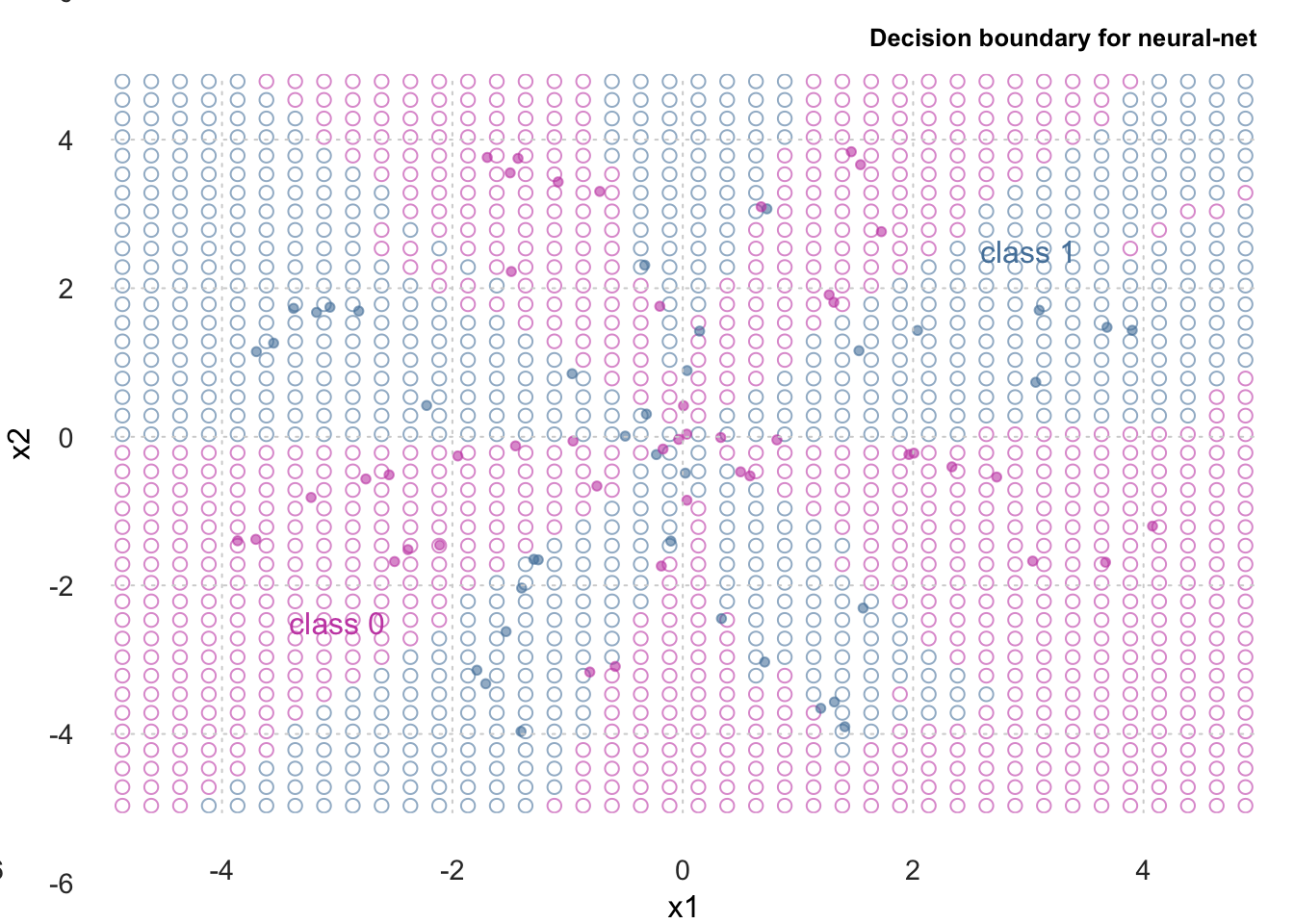#### Logistic Regression

On the other hand, Logistic Regression with it’s linear decision boundary could not fit the data very well.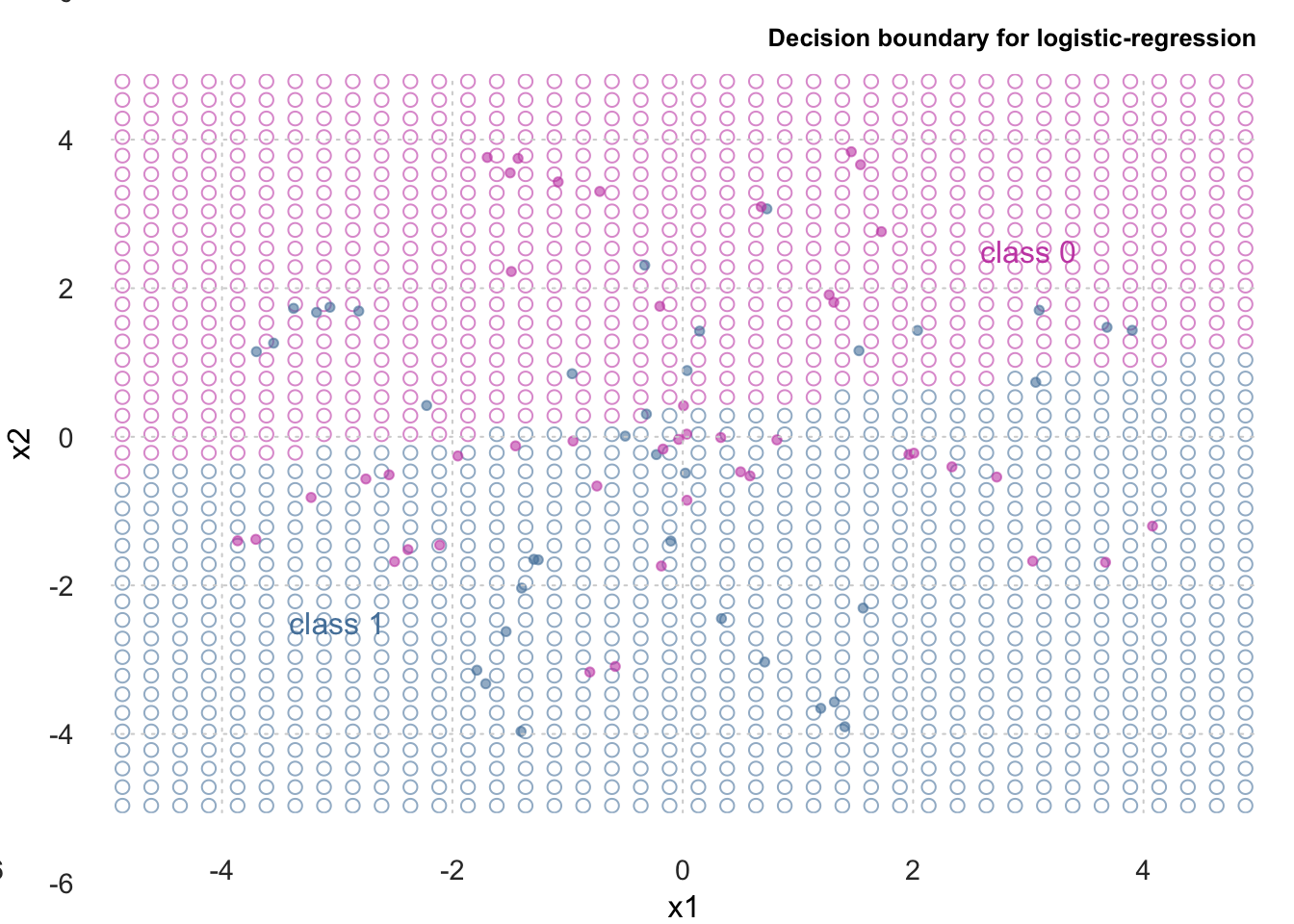### Confusion Matrix

A confusion matrix is often used to describe the performance of a classifier. It is defined as:

$\mathbf{Confusion Matrix} = \left[\begin{array} {rr} True Negative & False Positive \\ False Negative & True Positive \end{array}\right]$

Let’s go over the basic terms used in a confusion matrix through an example. Consider the case where we were trying to predict if an email was spam or not.

• True Positive: Email was predicted to be spam and it actually was spam.
• True Negative: Email was predicted as not-spam and it actually was not-spam.
• False Positive: Email was predicted to be spam but it actually was not-spam.
• False Negative: Email was predicted to be not-spam but it actually was spam.
tb_nn <- table(y_test, y_pred)
tb_lr <- table(y_test, lr_pred)

cat("NN Confusion Matrix: \n")
## NN Confusion Matrix:
tb_nn
##       y_pred
## y_test  0  1
##      0 34 10
##      1  7 29
cat("\nLR Confusion Matrix: \n")
##
## LR Confusion Matrix:
tb_lr
##       lr_pred
## y_test  0  1
##      0 14 30
##      1 18 18

### Accuracy Metrics

We’ll calculate the Precision, Recall, F1 Score, Accuracy. These metrics, derived from the confusion matrix, are defined as -

Precision is defined as the number of true positives over the number of true positives plus the number of false positives.

$Precision = \frac {True Positive}{True Positive + False Positive}$

Recall is defined as the number of true positives over the number of true positives plus the number of false negatives.

$Recall = \frac {True Positive}{True Positive + False Negative}$

F1-score is the harmonic mean of precision and recall.

$F1 Score = 2 \times \frac {Precision \times Recall}{Precision + Recall}$

Accuracy gives us the percentage of the all correct predictions out total predictions made.

$Accuracy = \frac {True Positive + True Negative} {True Positive + False Positive + True Negative + False Negative}$

To better understand these terms, let’s continue the example of “email-spam” we used above.

• If our model had a precision of 0.6, that would mean when it predicts an email as spam, then it is correct 60% of the time.

• If our model had a recall of 0.8, then it would mean our model correctly classifies 80% of all spam.

• The F-1 score is way we combine the precision and recall together. A perfect F1-score is represented with a value of 1, and worst with 0

Now that we have an understanding of the accuracy metrics, let’s actually calculate them. We’ll define a function that takes as input the confusion matrix. Then based on the above formulas, we’ll calculate the metrics.

calculate_stats <- function(tb, model_name) {
acc <- (tb + tb)/(tb + tb + tb + tb)
recall <- tb/(tb + tb)
precision <- tb/(tb + tb)
f1 <- 2 * ((precision * recall) / (precision + recall))

cat(model_name, ": \n")
cat("\tAccuracy = ", acc*100, "%.")
cat("\n\tPrecision = ", precision*100, "%.")
cat("\n\tRecall = ", recall*100, "%.")
cat("\n\tF1 Score = ", f1*100, "%.\n\n")
}

Here are the metrics for our neural-net and logistic regression.

## Neural Network :
##  Accuracy =  78.75 %.
##  Precision =  80.56 %.
##  Recall =  74.36 %.
##  F1 Score =  77.33 %.
## Logistic Regression :
##  Accuracy =  40 %.
##  Precision =  50 %.
##  Recall =  37.5 %.
##  F1 Score =  42.86 %.

As we can see, the logistic regression performed horribly because it cannot learn non-linear boundaries. Neural-nets on the other hand, are able to learn non-linear boundaries and as a result, have fit our complex data very well.

## Conclusion

In this two-part series, we’ve built a neural net from scratch with a vectorized implementation of backpropagation. We went through the entire life cycle of training a model; right from data pre-processing to model evaluation. Along the way, we learned about the mathematics that makes a neural-network. We went over basic concepts of linear algebra and calculus and implemented them as functions. We saw how to initialize weights, perform forward propagation, gradient descent, and back-propagation.

We learned about the ability of a neural net to fit to non-linear data and understood the importance of the role activation functions play in it. We trained a neural net and compared it’s performance to a logistic-regression model. We visualized the decision boundaries of both these models and saw how a neural-net was able to fit better than logistic regression. We learned about metrics like Precision, Recall, F1-Score, and Accuracy by evaluating our models against them.

You should now have a pretty solid understanding of how neural-networks are built.

I hope you had as much fun reading as I had while writing this! If I’ve made a mistake somewhere, I’d love to hear about it so I can correct it. Suggestions and constructive criticism are welcome. :)